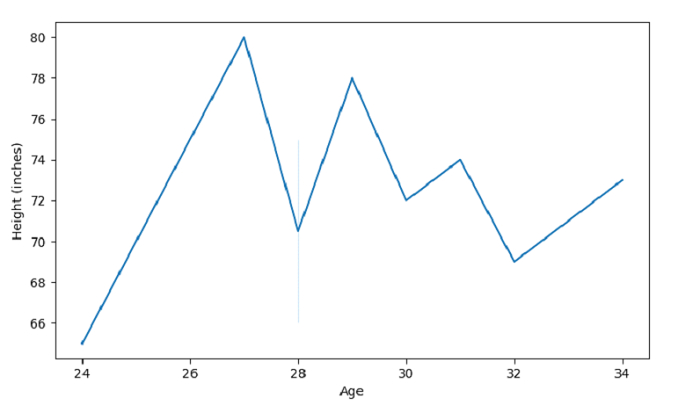# Plot a lineplot with Seaborn â€“ Python Pandas

Lineplot in Seaborn is used to draw a line plot with possibility of several semantic groupings. The seaborn.lineplot() is used for this.

Let’s say the following is our dataset in the form of a CSV file − Cricketers.csv

At first, import the required 3 libraries −

import seaborn as sb
import pandas as pd
import matplotlib.pyplot as plt

Load data from a CSV file into a Pandas DataFrame −

dataFrame = pd.read_csv("C:\Users\amit_\Desktop\Cricketers.csv")


## Example

Following is the code −

import seaborn as sb
import pandas as pd
import matplotlib.pyplot as plt

# Load data from a CSV file into a Pandas DataFrame

# Age in kgs
x = dataFrame['Age']

# Height in Inches
y = dataFrame['Height']

# plot lineplot with Age and Height
sb.lineplot(x,y)
plt.ylabel("Height (inches)")
plt.show()

## Output

This will produce the following output −